# Noise Suppression Products/EMI Suppression Filters

Noise Suppression Basic Course Section 1
Chapter 1
Chapter 2
Chapter 3
Chapter 4
Chapter 5
Chapter 6
Chapter 6

## EMI suppression filters

#### 6-5-2. Capacitor equivalent circuits

##### (1) Impedance at high frequencies tends to gather around the same value

Looking closely at the graph in Fig. 4 shows that the right side (high frequency side) of the V-shaped curves tend to gather at around the same location, regardless of the capacitor.
Fig. 5 overlays the impedance for an inductance of 0.5nH (dotted line) on the graph from Fig. 4 for comparison. Strangely enough, the measured impedance of the capacitor (right side of the V-shaped curve) is collected roughly on this line. In other words, the capacitor measured here (MLCC) shows an inductance of around 0.5nH at high frequencies.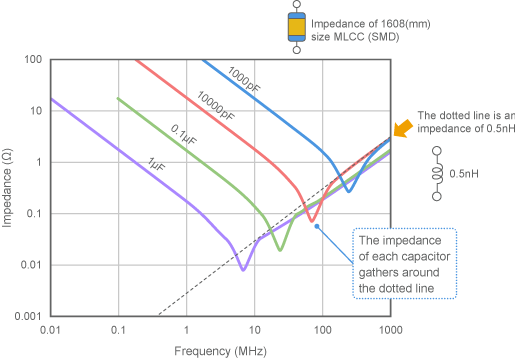Fig. 5 Relationship between capacitor impedance and ESL

##### (2) Equivalent circuits that take ESL into account

This inductance is referred to as the capacitor's ESL (Equivalent Series Inductance) . To represent a capacitor with ESL in an equivalent circuit, ESL is connected in series to the electrostatic capacitance (Cap) , as shown in Fig. 6.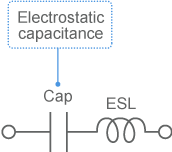Fig. 6 Capacitor equivalent circuit that takes only ESL into account

Note that, while an ESL of 0.5nH was used in Fig. 5, this value will vary depending on the capacitor. MLCCs of the same size were used in Fig. 5, so the ESL was around the same value. Using different capacitors will result in significantly different values.

##### (3) Self-resonance

As mentioned previously, capacitor impedance often forms a characteristic V-shaped curve, so the minimum point is in the central portion of the curve. This property is referred to as the capacitor's self-resonance; this is explained as the series resonance occurring between Cap and ESL in the equivalent circuit from Fig. 6. The frequency at the minimum point is called the SRF (self-resonant frequency) .
Incidentally, calculating the impedance using the equivalent circuit shown in Fig. 6 results in an impedance of zero at the self-resonant frequency. In other words, an actual capacitor can express a smaller impedance than the ideal capacitor at this frequency.

##### (4) ESR

Of course, an actual capacitor will exhibit a minor amount of loss, so the impedance will not be exactly zero even at the self-resonant frequency. In order to represent this loss, the ESR (Equivalent Series Resistance) , which is the amount of resistance, is normally included in capacitor equivalent circuits, as shown in Fig. 7.
The smaller the ESR is, the smaller the loss will be, and the better the capacitor will function. Fig. 8 shows an example of the impedance values of MLCCs with different ESRs. This shows that the self-resonant frequency impedance of a temperature compensation capacitor used in a resonant circuit is much smaller that of a general-purpose high-dielectric constant capacitor. This is because a capacitor with temperature compensation characteristics has a lower ESR.
Note that when a capacitor is self-resonating, its impedance demonstrates the ESR value of the capacitor. This is because the Cap and ESL impedance cancel each other out to zero in Fig. 7.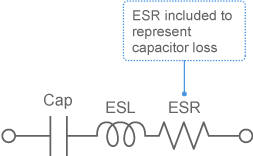Fig. 7 Capacitor equivalent circuit that takes ESL and ESR into account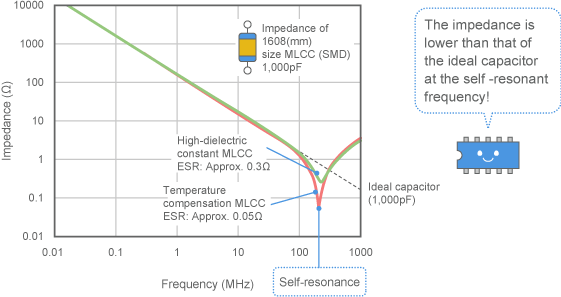Fig. 8 Example of capacitors with different ESR

##### (5) Impedance properties demonstrated by equivalent circuits

Fig. 9 (a) summarizes what has been covered thus far.
The impedance at the lower frequencies is roughly the same as that of an ideal capacitor. This is because the electrostatic capacitance impedance accounts for much of the total, so the effect of ESL and ESR can be ignored. A capacitor in this state is said to be "capacitive," and its impedance is inversely proportional to the frequency and electrostatic capacitance.
The impedance at the higher frequencies is roughly the same as the ESL impedance. This is because the ratio of this impedance is larger at higher frequencies. The capacitor is said to be "inductive" in this state, and the impedance is proportional to the frequency.
The self-resonant frequency is the region where the capacitor switches from capacitive to inductive, and it is also the minimum point of impedance. The impedance in this state is equivalent to ESR.
As an example, Fig. 9 (b) shows the result of calculating the impedance with representative values for a 0.1uF MLCC inserted in an equivalent circuit. As shown in (a) , the overall impedance follows the impedance of each element.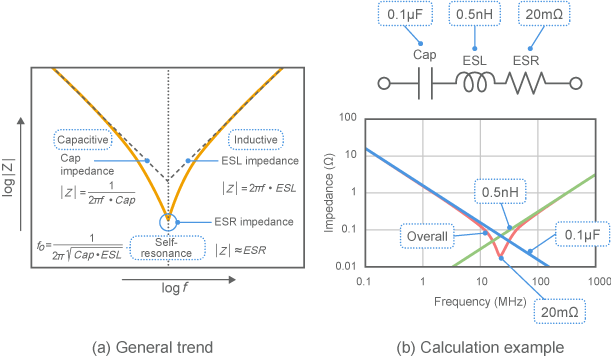Fig. 9 Capacitor impedance frequency characteristics

##### (6) How trustworthy are equivalent circuits?

Fig. 10 shows the actual measurements of a 1608 size MLCC overlapped with the example calculations from Fig. 9 (b) . The figure shows that, even when calculated using relatively approximate constants, the LCR series equivalent circuit shown in Fig. 7 closely matches the measured values and can reproduce the actual characteristics.
Note that, in order to reproduce actual characteristics more accurately, the ESR and ESL will need to be changed according to the frequency. Also, an MLCC was used for explanatory purposes here, this can also apply to other types of capacitors if the ESL and ESR values are adjusted.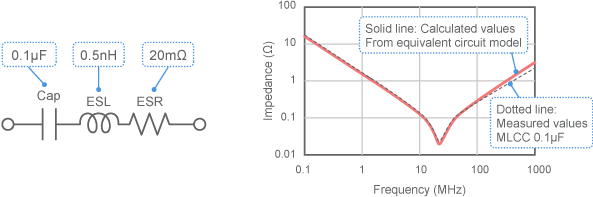Fig. 10 Comparison of calculated and measured values

##### (7) What causes ESL and ESR?

As shown previously, a capacitor's impedance is affected not only by the electrostatic capacitance, but also—at high frequencies—by ESL and ESR. These are referred to as "parasitic elements." In many cases they will reduce the noise reduction effect of the capacitor. What should be done to reduce these parasitic elements?
As shown in Fig. 11, an MLCC is composed of external and internal electrodes and dielectric bodies. The main factors behind ESR are the resistance of these external and internal electrodes, and dielectric body loss. Also, when electric current flows to the external and internal electrodes, a magnetic field is formed around the capacitor. This magnetic field is the main factor behind ESL.
Although reducing the effect of these parasitic elements is not an easy task, a capacitor with a reduced ESL used as an EMC measure will be introduced in a later chapter. These parasitic elements also occur when a capacitor is installed on a print board (this will be discussed later) . Capacitors must be installed with care so as to reduce ESL.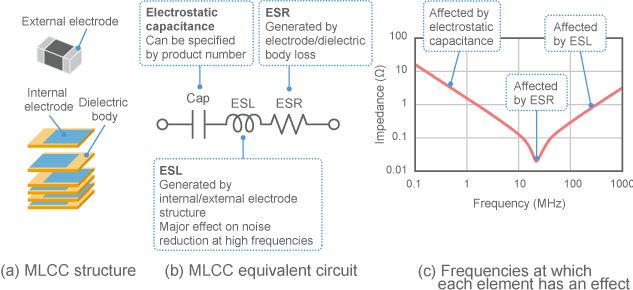Fig. 11 MLCC structure and equivalent circuit

Top of page